Author: 小小梦, Created: 2023-07-04 16:35:42, Updated: 2023-09-18 19:32:51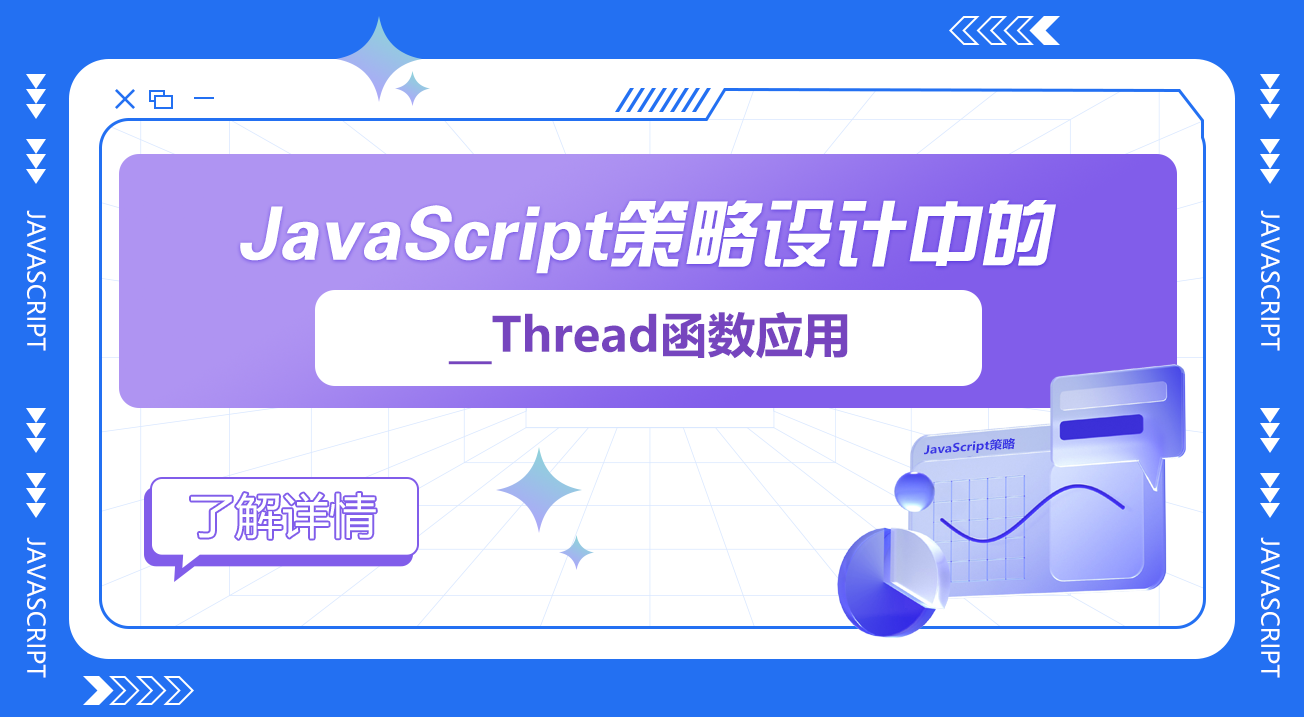### 1、简单的并发设计

``````__threadSetData(0, "ticker", t)
``````

``````__Thread(GetTickerAsync, 0)   // GetTickerAsync为需要并发执行的自定义函数，0为这个传入GetTickerAsync函数的参数
``````

``````var t = __threadGetData(0, "ticker")
Log(t)
``````

``````function GetTickerAsync(index) {
while (true) {
var t = exchanges[index].GetTicker()
Sleep(500)
}
}

function main() {

while(true) {
Log(t)
Sleep(1000)
}
}
``````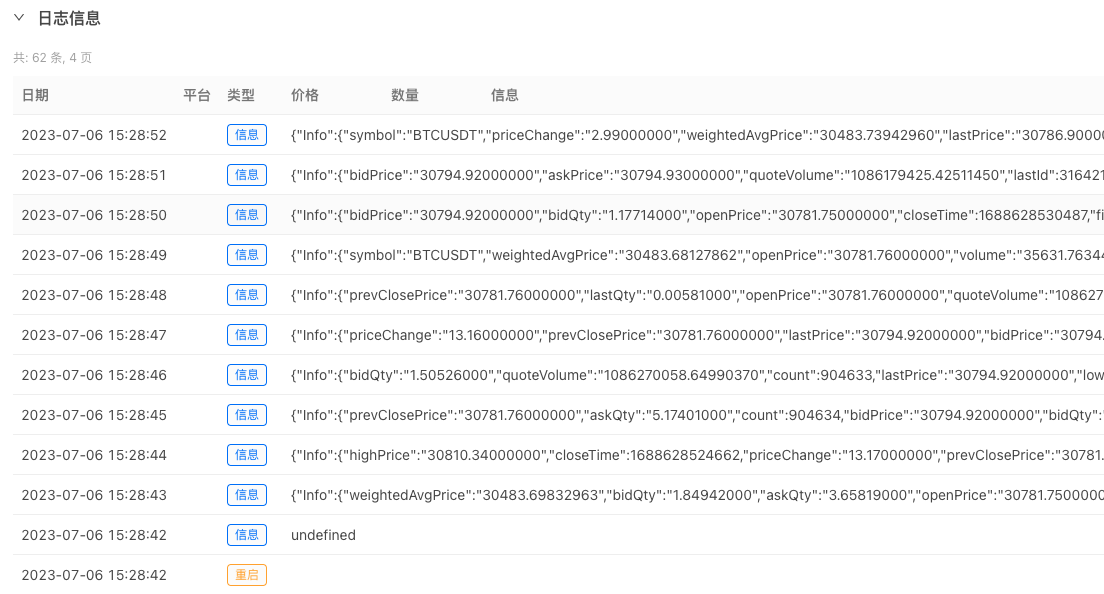### 2、并发下单设计

``````if (cmd == "placeMultipleOrders") {
// ...
}
``````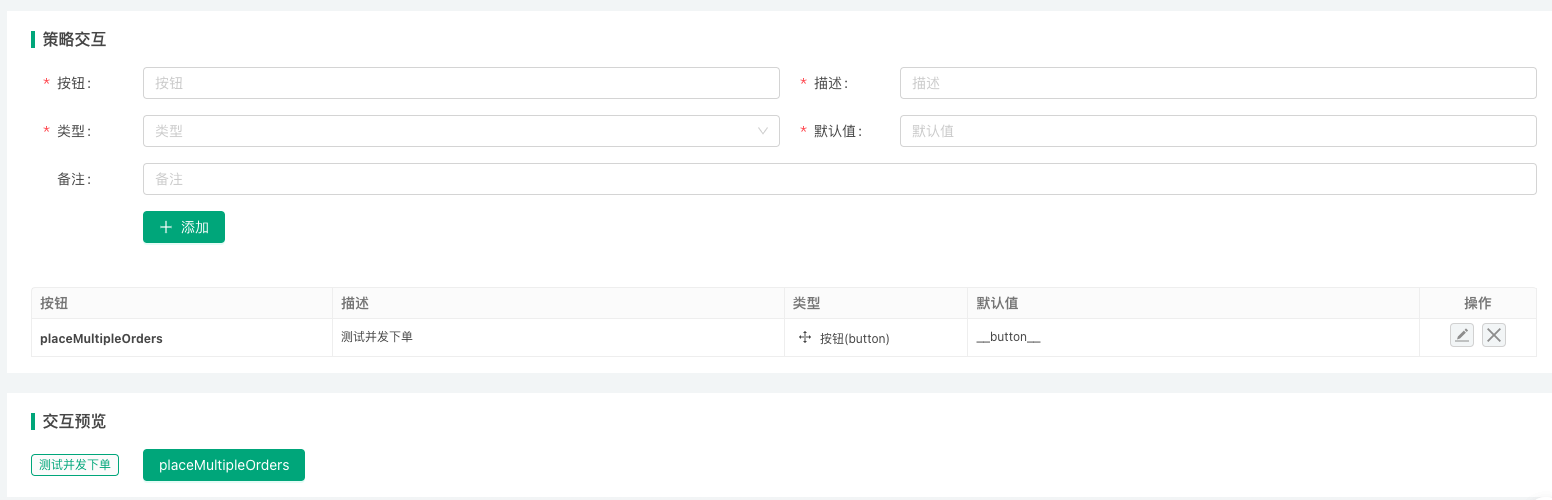``````function placeOrder(exIndex, type, price, amount) {
var id = null
} else if (type == "Sell") {
id = exchanges[exIndex].Sell(price, amount)
} else {
throw "type error! type:" + type
}
}

function testPlaceMultipleOrders(index, beginPrice, endPrice, step, type, amount) {
Log("beginPrice:", beginPrice, ", endPrice:", endPrice, ", step:", step, ", type:", type, ", amount:", amount)
var tids = []
for (var p = beginPrice; p <= endPrice; p += step) {
var tid = __Thread(placeOrder, index, type, p, amount)
tids.push(tid)
Sleep(10)
}
Sleep(1000)
for (var i = 0; i < tids.length; i++) {
}
}

function main() {
while(true) {
LogStatus(_D())
var cmd = GetCommand()
if (cmd) {
if (cmd == "placeMultipleOrders") {
var t = _C(exchange.GetTicker)
var beginPrice = t.Last * 0.8
var endPrice = t.Last * 0.9
var step = t.Last * 0.01
testPlaceMultipleOrders(0, beginPrice, endPrice, step, "Buy", 0.01)
var orders = exchange.GetOrders()
for (var i = 0; i < orders.length; i++) {
Log(orders[i])
}
}
}
Sleep(1000)
}
}
``````
• 测试使用挂单的方式，从当前价格80% ~ 90%递增，使用模拟盘环境测试，点击交互按钮触发测试下单：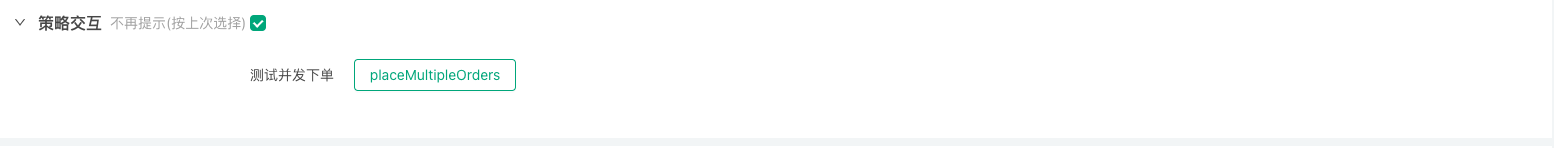• 点击「placeMultipleOrders」按钮后，提示信息：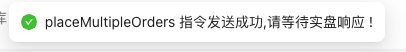• 策略日志上显示并发下单操作：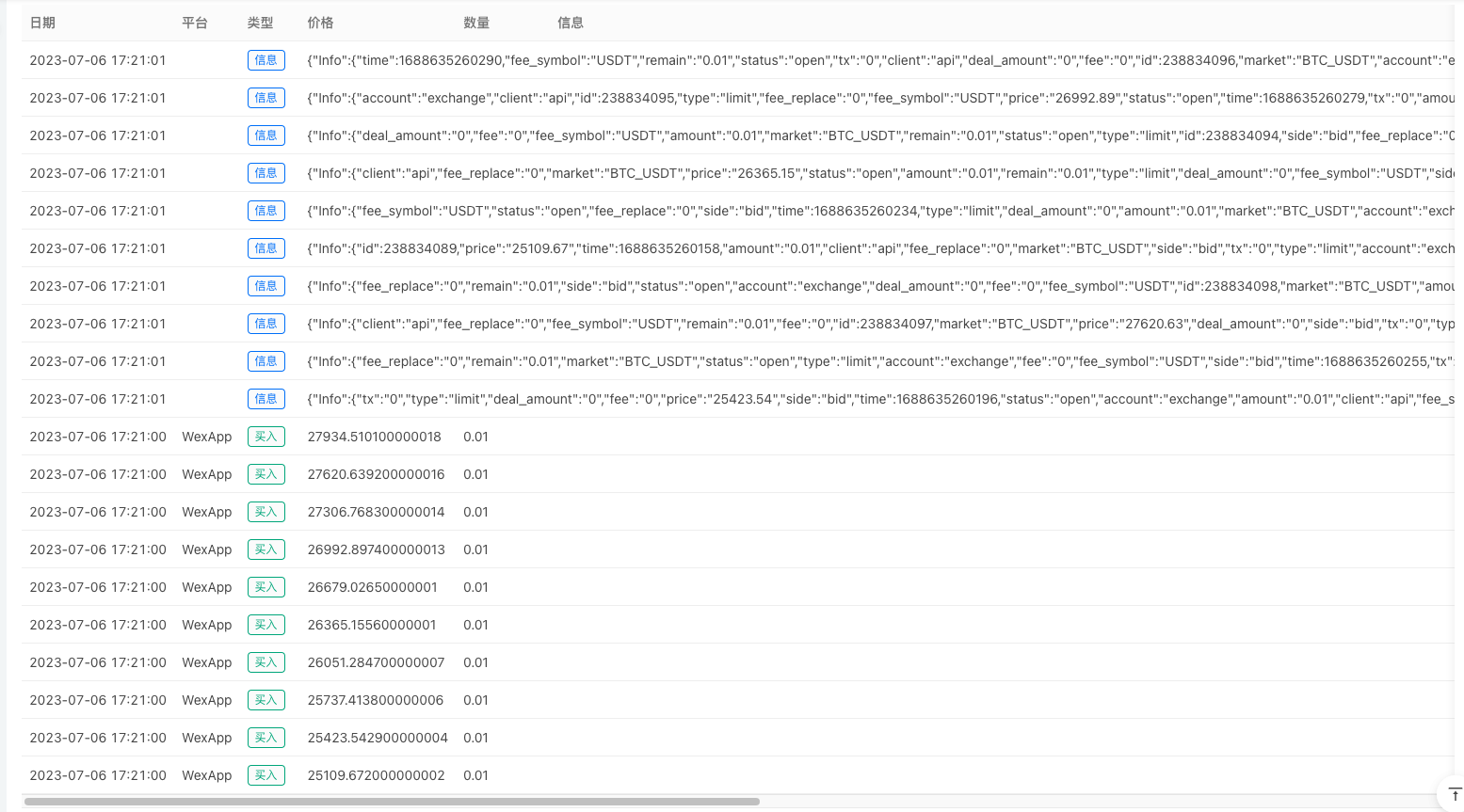### 3、在并发的线程执行函数中创建WebSocket连接

``````var tid = null

function createWS() {
// wss://stream.binance.com:9443/ws/<streamName> , <symbol>@ticker

var stream = "wss://stream.binance.com:9443/ws/btcusdt@ticker"
var ws = Dial(stream)
Log("创建WS连接:", stream)

while (true) {
if (data) {
}
Log("接收到WS链接推送的数据，data:", data)

if (msg) {
if (msg == "stop") {
break
}
}
}

Log("并发线程执行完毕，关闭ws连接")
ws.close()
}

function main() {
Log("创建并发线程，线程Id:", tid)

while(true) {
Log("接收到并发线程", ", Id:", tid, ", 发送的数据，data:", data, "#FF0000")

var tbl = {
type : "table",
title : "<symbol>@ticker频道推送消息",
cols : ["事件类型", "事件时间", "交易对", "24小时价格变化", "24小时价格变化百分比", "平均价格", "最新成交价格", "24小时内成交量", "24小时内成交额"],
rows : []
}

try {
data = JSON.parse(data)
tbl.rows.push([data.e, _D(data.E), data.s, data.p, data.P, data.w, data.c, data.v, data.q])
} catch (e) {
Log("e.name:", e.name, "e.stack:", e.stack, "e.message:", e.message)
}
LogStatus(_D(), "\n`" + JSON.stringify(tbl) + "`")
}
}

function onexit() {
Log("扫尾函数，向Id为", tid, "的并发线程发送stop指令")
Log("等待Id为", tid, "的并发线程停止")
Log("扫尾函数执行完毕")
}
``````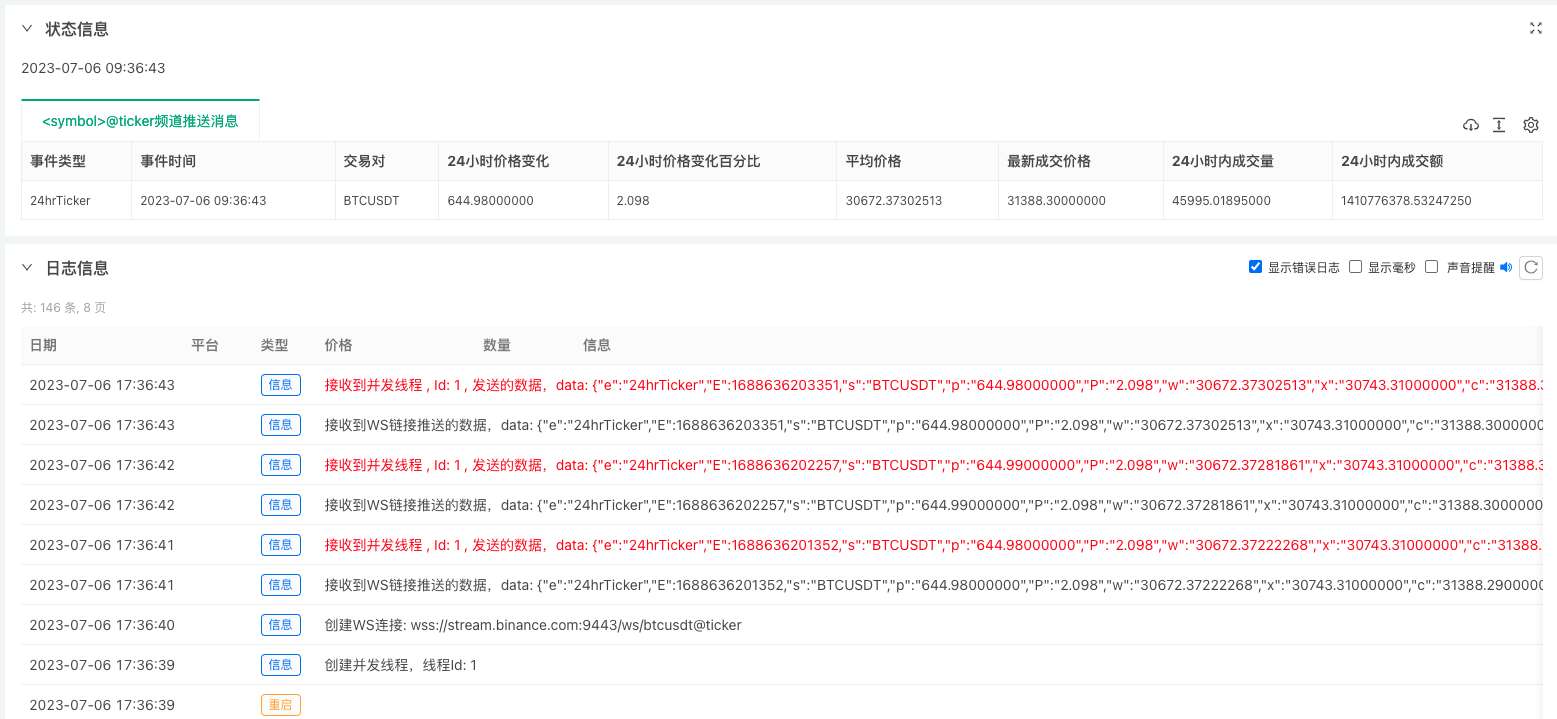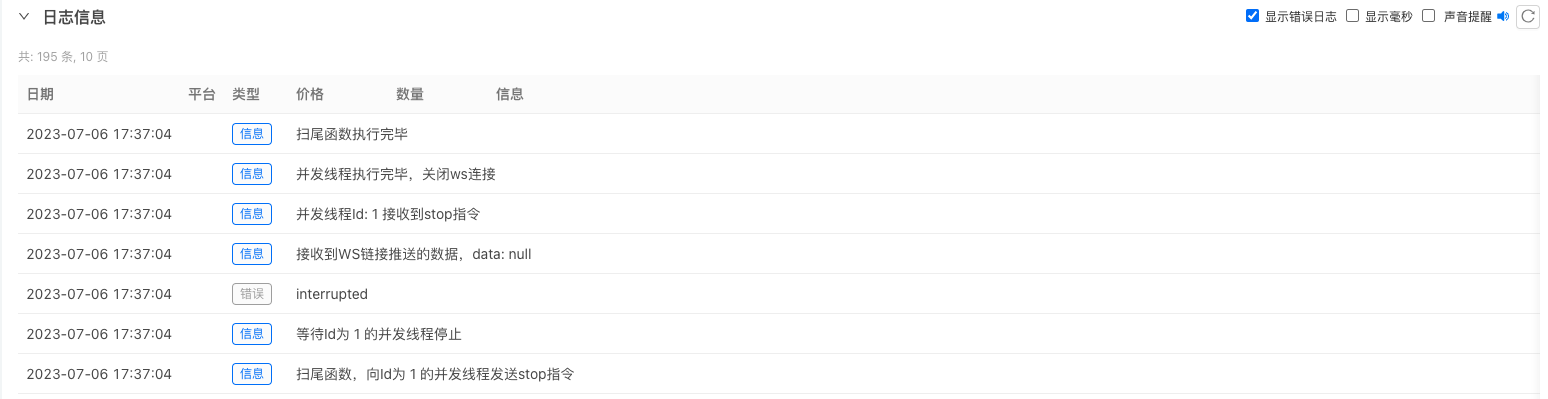Related

More